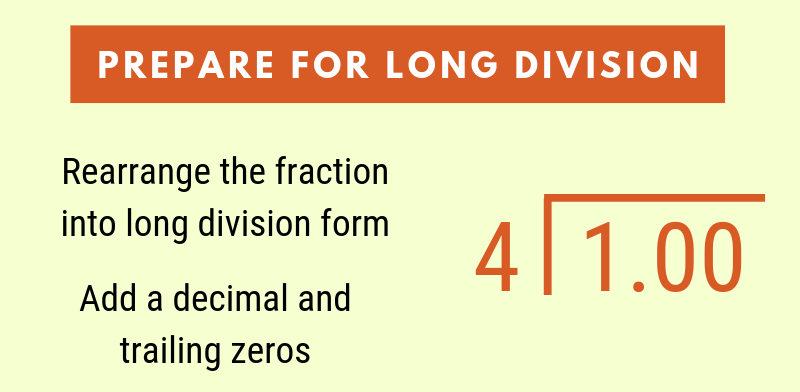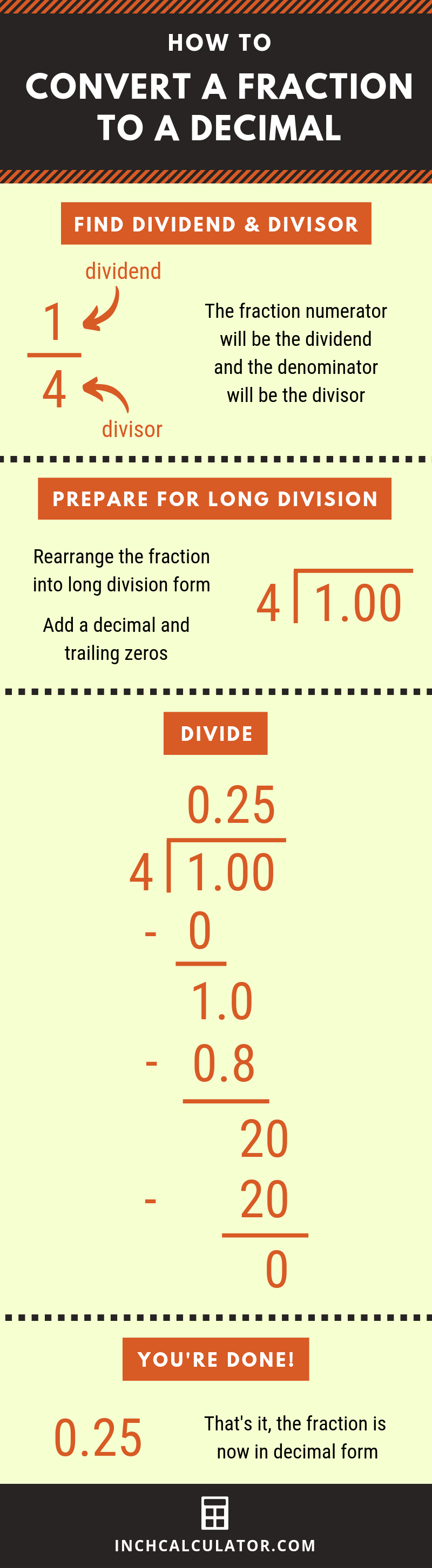# Fraction to Decimal Calculator

Convert a fraction to a decimal by entering your fraction below. See three methods to turn a fraction into a decimal below.

Fraction:

## Decimal Result:

5 / 2=2.5

### Steps to Convert Fraction to Decimal

Find the decimal by dividing the numerator by the denominator
5 / 2=5÷2=2.5
Learn how we calculated this below

Do you want to convert a decimal to a fraction?

## How to Convert a Fraction to Decimal

A number can be expressed as a fraction, decimal, or percentage, and sometimes it’s necessary to convert between the different forms to represent a number differently.

There are several methods to convert a fraction to a decimal; we’ll cover each of them below.

### Method 1: Convert a Fraction to Decimal Using a Calculator

The simplest method to transform a fraction number to a decimal value is to simply divide the numerator by the denominator to get the decimal value. The numerator is the top number, and the denominator is the bottom.

#### Example: Find 1/8 as a Decimal

Let’s convert the fraction 1/8 to a decimal using the division method.

1 / 8 = 1 ÷ 8 = 0.125

Thus, the decimal value of 1/8 is 0.125

You might also be interested in our fraction to percent calculator for similar conversions.

### Method 2: Convert a Fraction to Decimal Using Long Division

Long division offers another way to convert to decimal form. This is done by identifying the dividend and the divisor, then use those values in long division.

First, find the dividend and the divisor. The fraction’s numerator will be the dividend, and the denominator will be the divisor.Next, represent the dividend and divisor in long division form. It will likely be necessary to add a decimal and zeros if the dividend is smaller than the divisor.Finally, solve the long division problem to complete the conversion from fraction to decimal.Here’s a tip: use a long division calculator to solve this problem and see each step.

### Method 3: Convert a Fraction to Decimal by Simplifying

An alternate method to convert a fraction to a decimal number is to simplify it by putting the numerator over 1. This requires a few steps.

First, multiply the denominator to get to 100. To do this, try dividing 100 by the denominator to find the multiple, then multiply both the numerator and denominator by the multiple.

The final step is to move the decimal place on the new numerator to the left two places to change the fraction to its decimal value.

#### Example: 1/16 to Decimal

For example, we can convert the fraction 1/16 to a decimal value using this method.

Start by finding the multiple needed to multiply the denominator by to get 100.

100 = 16 × 6.25

That means that the multiple is 6.25.

Now, multiple the numerator by the multiple (6.25).

1 × 6.25 = 6.25

Thus, the fraction 1/16 can also be represented as 6.25/100.

1 / 16 = 6.25 / 100

Finally, move the numerator’s decimal place to the left two places to get the decimal value.

6.25 -> 0.0625

So, the decimal value of 1/16 is 0.0625.

## Fraction to Decimal Conversion Chart

Another way to convert fractions to decimals is to consult a conversion chart, such as the one below, which shows the decimal values of a few common fractions.

Fraction to decimal conversion chart showing common fractions and their equivalent decimal values. The chart shows fractions with denominators up to 20.
Fraction Decimal
1/2 0.5
1/3 0.3333
2/3 0.6667
1/4 0.25
3/4 0.75
1/5 0.2
2/5 0.4
3/5 0.6
4/5 0.8
1/6 0.1667
5/6 0.8333
1/7 0.1429
2/7 0.2857
3/7 0.4286
4/7 0.5714
5/7 0.7143
6/7 0.8571
1/8 0.125
3/8 0.375
5/8 0.625
7/8 0.875
1/9 0.1111
2/9 0.2222
4/9 0.4444
5/9 0.5556
7/9 0.7778
8/9 0.8889
1/10 0.1
3/10 0.3
7/10 0.7
9/10 0.9
1/11 0.0909
2/11 0.1818
3/11 0.2727
4/11 0.3636
5/11 0.4545
6/11 0.5455
7/11 0.6364
8/11 0.7273
9/11 0.8182
10/11 0.9091
1/12 0.0833
5/12 0.4167
7/12 0.5833
11/12 0.9167
1/13 0.0769
2/13 0.1538
3/13 0.2308
4/13 0.3077
5/13 0.3846
6/13 0.4615
7/13 0.5385
8/13 0.6154
9/13 0.6923
10/13 0.7692
11/13 0.8462
12/13 0.9231
1/14 0.0714
3/14 0.2143
5/14 0.3571
9/14 0.6429
11/14 0.7857
13/14 0.9286
1/15 0.0667
2/15 0.1333
4/15 0.2667
7/15 0.4667
8/15 0.5333
11/15 0.7333
13/15 0.8667
14/15 0.9333
1/16 0.0625
3/16 0.1875
5/16 0.3125
7/16 0.4375
9/16 0.5625
11/16 0.6875
13/16 0.8125
15/16 0.9375
1/17 0.0588
2/17 0.1176
3/17 0.1765
4/17 0.2353
5/17 0.2941
6/17 0.3529
7/17 0.4118
8/17 0.4706
9/17 0.5294
10/17 0.5882
11/17 0.6471
12/17 0.7059
13/17 0.7647
14/17 0.8235
15/17 0.8824
16/17 0.9412
1/18 0.0556
5/18 0.2778
7/18 0.3889
11/18 0.6111
13/18 0.7222
17/18 0.9444
1/19 0.0526
2/19 0.1053
3/19 0.1579
4/19 0.2105
5/19 0.2632
6/19 0.3158
7/19 0.3684
8/19 0.4211
9/19 0.4737
10/19 0.5263
11/19 0.5789
12/19 0.6316
13/19 0.6842
14/19 0.7368
15/19 0.7895
16/19 0.8421
17/19 0.8947
18/19 0.9474
1/20 0.05
3/20 0.15
7/20 0.35
9/20 0.45
11/20 0.55
13/20 0.65
17/20 0.85
19/20 0.95

See our inch fraction calculator for a comprehensive inch fraction to decimal chart.

You’ll probably also find our equivalent fraction chart useful.